# Making Asteroids with Kontra.js and Web Maker

Making games for the first time can always be a daunting task. So many things to learn and understand. But don’t worry, I’ll help you through your first game. Also, the Js13kGames game jam is starting soon. So what better time to learn how to make a game!

In this tutorial, we’ll make the classic arcade game Asteroids. You can read more about Asteroids if you’re not familiar with the game.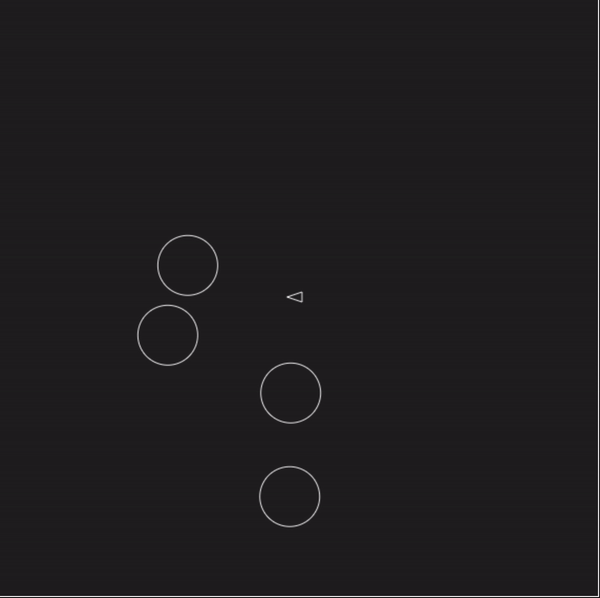Asteroids game we’ll be making

# Use a Library

We’ll be using the Kontra.js game library. It’s a lightweight library I built for the JS13kGames game jam.

What’s nice about using a library for your first game is that it takes care of all the hard work for you. Instead of worrying about how to set up the game loop or manage all the sprites, you can just focus on the game itself.

For this game, we’re just going to make a simplified version of Asteroids. Just asteroids, the player ship, and bullets. This will make it easier to get going and you can always add more to it.

# Setting up the Game With Web Maker

We’ll be using Web Maker app as it makes it convenient to set up a Kontra.js game. Fire it up - https://webmaker.app/app/

First, if you are participating in the Js13kGames compo, turn on the Js13kGames Mode from the settings to set the right gamedev environment :)

Now click the New button at the top of the screen. Web Maker provides you with a few different templates to get you up and running quickly. From the list of Templates, select Kontra Game Engine to prepopulate the editor with a Kontra.js project.

Click the Add Library button and change the JS file to use the latest version of Kontra.js file (e.g. https://unpkg.com/kontra@latest/kontra.js).

Next, expand the HTML section of the editor and update the canvas element with a width and height so we have a larger game to play on.

`<canvas width="600" height="600"></canvas>`

We’ll also want the background to be black and the game to have a white border so we know where the edges of screen are. In the CSS section of the editor add this:

`body {  background: black;}canvas {  border: 1px solid white;  } `

The last thing to do is delete everything but the first line from the JS section of the editor. The template provides you with some starter code so you can get familiar with using Kontra.js. Since we’ll be making our own game we can go ahead and remove most of it and leave just the initialization of the library using kontra.init().

kontra.init() returns the `canvas` and `context` used for the game, so we’ll go ahead and save those for later use by destructuring the return object.

`let { canvas, context } = kontra.init();`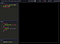Web Maker app with initial code

# Creating an Asteroid

Now that everything’s set up, we can start making the game. Let’s start by making an asteroid game object.

To create a game object, or sprite, in Kontra we use kontra.Sprite() and pass it any information we want.

For our asteroid, we’ll pass it the x and y position, the speed of the asteroid (`dx` and `dy`), and a `render()` function to tell the sprite how to draw the asteroid. In this case, the asteroid will be just a circle.

Notice that we draw the circle using the coordinates `{0, 0}` . This is because Kontra will automatically move the origin of the canvas to the x and y position of the sprite. This means all drawing coordinates should be relative to the sprites position.

`let asteroid = kontra.Sprite({  type: 'asteroid',  // we'll use this later for collision detection  x: 100,  y: 100,  dx: Math.random() * 4 - 2,  dy: Math.random() * 4 - 2,  radius: 30,  render() {    this.context.strokeStyle = 'white';    this.context.beginPath();  // start drawing a shape    this.context.arc(0, 0, this.radius, 0, Math.PI*2);    this.context.stroke();     // outline the circle  }});asteroid.render();`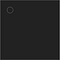A lone, non-moving asteroid

# Creating the Game Loop

To move the sprite, we’ll need a game loop to update and render it every frame. Game loop’s `update` and `render` are simply functions that the game engine calls in a loop at right times for you. We’ll create a kontra.GameLoop() and pass it `update()` and `render()` functions to update and render the sprite.

Right afterwards, we’ll call loop.start() to start the game loop.

`let loop = kontra.GameLoop({  update() {    asteroid.update();  },  render() {    asteroid.render();  }});loop.start();`

# Wrapping Around the Screen

The asteroid should start moving. But when it reaches the edges it’ll keep going off the screen. Instead, we want the asteroid to wrap around the edges.

To do that, we’ll modify the `update()` function to check the position of the asteroid after we've updated it and see if it's beyond the edges of the screen. If it is, we'll move it to the opposite edge.

We can check the dimensions of the game by using the canvas element we saved earlier to access the canvases `width` and `height` properties.

Go ahead and update the game loop’s `update()` function. Note that we use the asteroid radius to determine if it is offscreen. This makes sure the asteroid is completely offscreen before moving it to the opposite edge.

`update() {  asteroid.update();    // asteroid is beyond the left edge  if (asteroid.x < -asteroid.radius) {    asteroid.x = canvas.width + asteroid.radius;  }  // asteroid is beyond the right edge  else if (asteroid.x > canvas.width + asteroid.radius) {    asteroid.x = 0 - asteroid.radius;  }  // asteroid is beyond the top edge  if (asteroid.y < -asteroid.radius) {    asteroid.y = canvas.height + asteroid.radius;  }  // asteroid is beyond the bottom edge  else if (asteroid.y > canvas.height + asteroid.radius) {    asteroid.y = -asteroid.radius;  }}`

# More Asteroids

We now have a moving asteroid. But just one isn’t enough, we need more for the player to shoot.

Since there’s going to be multiple asteroids, we should update the code to handle moving multiple sprites, including the ship and bullets. One way we can do that is to store all the sprites in an array and loop over it to update all the sprites.

We should also turn our asteroid creation code into a function so we can call it multiple times. The function should create an asteroid and then add it to the array.

At this point we should also add some randomness to the asteroids so they all don’t move in the same direction. We’ll want both negative numbers and positive numbers for the direction so they don’t always move to the right or bottom.

A range of `-2` to `2` seems like a good range and doesn't make the asteroids move too fast. To get this range, we can multiply `Math.random()` by four, then subtract by two.

If we make all the changes, the full game code should look like this:

`let { canvas, context } = kontra.init();let sprites = [];function createAsteroid() {  let asteroid = kontra.Sprite({    type: 'asteroid',  // we'll use this for collision detection    x: 100,    y: 100,    dx: Math.random() * 4 - 2,    dy: Math.random() * 4 - 2,    radius: 30,    render() {      this.context.strokeStyle = 'white';      this.context.beginPath();  // start drawing a shape      this.context.arc(0, 0, this.radius, 0, Math.PI*2);      this.context.stroke();     // outline the circle    }  });  sprites.push(asteroid);}for (let i = 0; i < 4; i++) {  createAsteroid();}let loop = kontra.GameLoop({  update() {    sprites.map(sprite => {      sprite.update();      // asteroid is beyond the left edge      if (sprite.x < -sprite.radius) {        sprite.x = canvas.width + sprite.radius;      }      // sprite is beyond the right edge      else if (sprite.x > canvas.width + sprite.radius) {        sprite.x = 0 - sprite.radius;      }      // sprite is beyond the top edge      if (sprite.y < -sprite.radius) {        sprite.y = canvas.height + sprite.radius;      }      // sprite is beyond the bottom edge      else if (sprite.y > canvas.height + sprite.radius) {        sprite.y = -sprite.radius;      }    });  },  render() {    sprites.map(sprite => sprite.render());  }});loop.start();`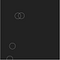Four Asteroids moving around the screen

# The Player Ship

It’s time to add the player ship. We’ll start by drawing a triangular sprite. Just as we did for the asteroid, we’ll pass it the position and `render()` function.

`let ship = kontra.Sprite({  x: 300,  y: 300,  radius: 6,  // we'll use this later for collision detection  render() {    // draw a right-facing triangle    this.context.strokeStyle = 'white';    this.context.beginPath();    this.context.moveTo(-3, -5);    this.context.lineTo(12, 0);    this.context.lineTo(-3, 5);    this.context.closePath();    this.context.stroke();  }});sprites.push(ship);`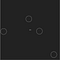Player ship surrounded by asteroids

# Rotating the Player Ship

Next we’ll make the ship move by allowing it to rotate.

When the player presses the left arrow key the ship should rotate left, and rotate right when the right arrow key is pressed. To do this, we’ll pass an `update()` function to the ship sprite and check if those keys are pressed using kontra.keyPressed(). However, before we do that we need to initialize the keyboard using kontra.initKeys().

Managing rotation can be tricky, but Kontra does all the heavy lifting for you. All you have to do is use the `rotation` property of the sprite and Kontra will automatically rotate it.

There are two things to be aware of when using `rotation`:

• Rotations are in in radians, not degrees. I find radians hard to work with so usually I work in degrees and convert it to radians. Kontra provides a helper function degToRad() to do this for you.
• Zero degrees is not up, it’s to the right. This is because zero radians starts at the right.

Let’s update the ships `update()` function to handle rotations.

`kontra.initKeys();let ship = kontra.Sprite({  x: 300,  y: 300,  radius: 6,  // we'll use this later for collision detection  render() {    // draw a right-facing triangle    this.context.strokeStyle = 'white';    this.context.beginPath();    this.context.moveTo(-3, -5);    this.context.lineTo(12, 0);    this.context.lineTo(-3, 5);    this.context.closePath();    this.context.stroke();  },  update() {    // rotate the ship left or right    if (kontra.keyPressed('left')) {      this.rotation += kontra.degToRad(-4);    }    else if (kontra.keyPressed('right')) {      this.rotation += kontra.degToRad(4);    }  }});`

# Ship Thrust

Now that we’ve done all the hard work to make the ship rotate, we can make the ship move.

When the player presses the up arrow key, the ship should move forward in the direction it’s facing.

We can do that by using Math.cos() and Math.sin() to get the x and y unit of the rotation (respectively). Then we can multiple each unit by how fast we want the ship to accelerate.

Each Kontra.js sprite uses `ddx` and `ddy` as the x and y values of the acceleration vector. To have the ship move based on its acceleration, we call ship.advance().

Update the ships `update()` function to move the ship forward.

`update() {  // rotate the ship left or right  if (kontra.keyPressed('left')) {    this.rotation += kontra.degToRad(-4);  }  else if (kontra.keyPressed('right')) {    this.rotation += kontra.degToRad(4);  }  // move the ship forward in the direction it's facing  const cos = Math.cos(this.rotation);  const sin = Math.sin(this.rotation);  if (kontra.keyPressed('up')) {    this.ddx = cos * 0.05;    this.ddy = sin * 0.05;  }  this.advance();}`

# Ship Maximum Speed

The ship should now move forward when you press the up arrow. However the ship will continually speed up even if you let go. Instead, we want the ship to stop accelerating when the player releases the up arrow key.

This can be achieved by setting the `ddx` and `ddy` to zero if the up arrow key is not pressed.

However, the ship can continuously speed up so long as the player presses the up arrow key. We should set a max speed so the ship doesn’t become uncontrollable.

To do this, we’ll need to check the magnitude of the `velocity` vector (`dx` and `dy`) and see if it’s greater than the max speed. If it is, we dial down the speed a bit. We can use vector.length() to get the magnitude.

Once again, update the ships `update()` function.

`update() {  // rotate the ship left or right  if (kontra.keyPressed('left')) {    this.rotation += kontra.degToRad(-4);  }  else if (kontra.keyPressed('right')) {    this.rotation += kontra.degToRad(4);  }  // move the ship forward in the direction it's facing  const cos = Math.cos(this.rotation);  const sin = Math.sin(this.rotation);  if (kontra.keyPressed('up')) {    this.ddx = cos * 0.05;    this.ddy = sin * 0.05;  }  else {    this.ddx = this.ddy = 0;  }  this.advance();  // set a max speed  if (this.velocity.length() > 5) {    this.dx *= 0.95;    this.dy *= 0.95;  }}`

# Firing Bullets

The last sprite to code is the bullets.

When the player presses the spacebar, the ship should fire a bullet in the direction the player is facing. However, we want to limit the amount of bullets the player can shoot so it’s not an infinite stream of bullets.

We can do this by keeping track of how much time has passed since the players last shot. If enough time has elapsed, we can fire another bullet by adding it to the sprites array.

Kontra.js guarantees a frame rate of 60FPS, so each frame is exactly 1/60 of a second. Using this, we can track how much time has passed in a new variable `dt`.

Each bullet should start at the end of the ship and move slightly faster than the ship is moving. Each bullet should also only live for a short time on the screen.

To do this, we’ll set the ttl (time to live) property on the bullet. This property tells the sprite how many frames it should be alive. We can then use array.filter() to remove any dead bullets from the array by checking sprite.isAlive().

The ship code should now look like this:

`let ship = kontra.Sprite({  x: 300,  y: 300,  radius: 6,  // we'll use this later for collision detection  dt: 0,  // track how much time has passed  render() {    // draw a right-facing triangle    this.context.strokeStyle = 'white';    this.context.beginPath();    this.context.moveTo(-3, -5);    this.context.lineTo(12, 0);    this.context.lineTo(-3, 5);    this.context.closePath();    this.context.stroke();  },  update() {    // rotate the ship left or right    if (kontra.keyPressed('left')) {      this.rotation += kontra.degToRad(-4);    }    else if (kontra.keyPressed('right')) {      this.rotation += kontra.degToRad(4);    }    // move the ship forward in the direction it's facing    const cos = Math.cos(this.rotation);    const sin = Math.sin(this.rotation);    if (kontra.keyPressed('up')) {      this.ddx = cos * 0.05;      this.ddy = sin * 0.05;    }    else {      this.ddx = this.ddy = 0;    }    this.advance();    // set a max speed    if (this.velocity.length() > 5) {      this.dx *= 0.95;      this.dy *= 0.95;    }    // allow the player to fire no more than 1 bullet every 1/4 second    this.dt += 1/60;    if (kontra.keyPressed('space') && this.dt > 0.25) {      this.dt = 0;      let bullet = kontra.Sprite({        color: 'white',        // start the bullet on the ship at the end of the triangle        x: this.x + cos * 12,        y: this.y + sin * 12,        // move the bullet slightly faster than the ship        dx: this.dx + cos * 5,        dy: this.dy + sin * 5,        // live only 50 frames        ttl: 50,        // bullets are small        radius: 2,        width: 2,        height: 2      });      sprites.push(bullet);    }  }});`

At the end of the game loop update code you can filter out dead bullets.

`sprites = sprites.filter(sprite => sprite.isAlive());`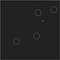Ship firing bullets at the asteroids

# Collision Detection

We’re almost done. Now that we have bullets we need to check for collision between the asteroids and the other objects.

We can do this inside the loops `update()` function. We're going to cheat a bit to keep things simple and just assume everything's a circle. Then we can use a simple circle vs. circle collision check.

Using the `type` property, we can determine which sprite are asteroids and then find all the non-asteroid sprites and check collision between them.

The `update()` function should now look like this:

`update() {  sprites.map(sprite => {    sprite.update();    // asteroid is beyond the left edge    if (sprite.x < -sprite.radius) {      sprite.x = canvas.width + sprite.radius;    }    // sprite is beyond the right edge    else if (sprite.x > canvas.width + sprite.radius) {      sprite.x = 0 - sprite.radius;    }    // sprite is beyond the top edge    if (sprite.y < -sprite.radius) {      sprite.y = canvas.height + sprite.radius;    }    // sprite is beyond the bottom edge    else if (sprite.y > canvas.height + sprite.radius) {      sprite.y = -sprite.radius;    }  });  // collision detection  for (let i = 0; i < sprites.length; i++) {    // only check for collision against asteroids    if (sprites[i].type === 'asteroid') {      for (let j = 0; j < sprites.length; j++) {        // don't check asteroid vs. asteroid collisions        if (sprites[j].type !== 'asteroid') {          let asteroid = sprites[i];          let sprite = sprites[j];          // circle vs. circle collision detection          let dx = asteroid.x - sprite.x;          let dy = asteroid.y - sprite.y;          if (Math.hypot(dx, dy) < asteroid.radius + sprite.radius) {            asteroid.ttl = 0;            sprite.ttl = 0;            break;          }        }      }    }  }  sprites = sprites.filter(sprite => sprite.isAlive());}`

# Splitting the Asteroid

With collision detection up and running, we can add the final bit of the game: splitting the asteroid into three smaller asteroids.

This requires updating our `createAsteroid()` function to be able to take an x and y position so we can create the asteroid where the previous one was. We'll also want to be able to pass it the size so we can create smaller asteroids.

Lastly we’ll need to update the first four calls to the function to pass it the new information.

Update the function and calls to the function like so:

`function createAsteroid(x, y, radius) {  let asteroid = kontra.Sprite({    type: 'asteroid',    x: x,    y: y,    dx: Math.random() * 4 - 2,    dy: Math.random() * 4 - 2,    radius: radius,    render() {      this.context.strokeStyle = 'white';      this.context.beginPath();  // start drawing a shape      this.context.arc(this.x, this.y, this.radius, 0, Math.PI*2);      this.context.stroke();     // outline the circle    }  });  sprites.push(asteroid);}for (var i = 0; i < 4; i++) {  createAsteroid(100, 100, 30);}`

Then in the game loop `update()` function, when there is a collision we can create three smaller asteroids in it's place. An asteroid can only split two times, so we'll check the radius of the asteroid to see if it's big enough to split.

`if (Math.hypot(dx, dy) < asteroid.radius + sprite.radius) {  asteroid.ttl = 0;  sprite.ttl = 0;  // split the asteroid only if it's large enough  if (asteroid.radius > 10) {    for (var x = 0; x < 3; x++) {      createAsteroid(asteroid.x, asteroid.y, asteroid.radius / 2.5);    }  }  break;}`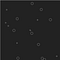An asteroid field of small asteroids after all the larger ones have split

# Game Over

Congratulations you’ve just made your first game! We now have a fully functional Asteroids game.

Here is the complete JavaScript code for reference:

`let { canvas, context } = kontra.init();let sprites = [];function createAsteroid(x, y, radius) {  let asteroid = kontra.Sprite({    type: 'asteroid',  // we'll use this for collision detection    x,    y,    dx: Math.random() * 4 - 2,    dy: Math.random() * 4 - 2,    radius,    render() {      this.context.strokeStyle = 'white';      this.context.beginPath();  // start drawing a shape      this.context.arc(0, 0, this.radius, 0, Math.PI*2);      this.context.stroke();     // outline the circle    }  });  sprites.push(asteroid);}for (let i = 0; i < 4; i++) {  createAsteroid(100, 100, 30);}kontra.initKeys();let ship = kontra.Sprite({  x: 300,  y: 300,  radius: 6,  // we'll use this later for collision detection  dt: 0,  // track how much time has passed  render() {    // draw a right-facing triangle    this.context.strokeStyle = 'white';    this.context.beginPath();    this.context.moveTo(-3, -5);    this.context.lineTo(12, 0);    this.context.lineTo(-3, 5);    this.context.closePath();    this.context.stroke();  },  update() {    // rotate the ship left or right    if (kontra.keyPressed('left')) {      this.rotation += kontra.degToRad(-4);    }    else if (kontra.keyPressed('right')) {      this.rotation += kontra.degToRad(4);    }    // move the ship forward in the direction it's facing    const cos = Math.cos(this.rotation);    const sin = Math.sin(this.rotation);    if (kontra.keyPressed('up')) {      this.ddx = cos * 0.05;      this.ddy = sin * 0.05;    }    else {      this.ddx = this.ddy = 0;    }    this.advance();    // set a max speed    if (this.velocity.length() > 5) {      this.dx *= 0.95;      this.dy *= 0.95;    }    // allow the player to fire no more than 1 bullet every 1/4 second    this.dt += 1/60;    if (kontra.keyPressed('space') && this.dt > 0.25) {      this.dt = 0;      let bullet = kontra.Sprite({        color: 'white',        // start the bullet on the ship at the end of the triangle        x: this.x + cos * 12,        y: this.y + sin * 12,        // move the bullet slightly faster than the ship        dx: this.dx + cos * 5,        dy: this.dy + sin * 5,        // live only 50 frames        ttl: 50,        // bullets are small        radius: 2,        width: 2,        height: 2      });      sprites.push(bullet);    }  }});sprites.push(ship);let loop = kontra.GameLoop({  update() {    sprites.map(sprite => {      sprite.update();      // asteroid is beyond the left edge      if (sprite.x < -sprite.radius) {        sprite.x = canvas.width + sprite.radius;      }      // sprite is beyond the right edge      else if (sprite.x > canvas.width + sprite.radius) {        sprite.x = 0 - sprite.radius;      }      // sprite is beyond the top edge      if (sprite.y < -sprite.radius) {        sprite.y = canvas.height + sprite.radius;      }      // sprite is beyond the bottom edge      else if (sprite.y > canvas.height + sprite.radius) {        sprite.y = -sprite.radius;      }    });    // collision detection    for (let i = 0; i < sprites.length; i++) {      // only check for collision against asteroids      if (sprites[i].type === 'asteroid') {        for (let j = 0; j < sprites.length; j++) {          // don't check asteroid vs. asteroid collisions          if (sprites[j].type !== 'asteroid') {            let asteroid = sprites[i];            let sprite = sprites[j];            // circle vs. circle collision detection            let dx = asteroid.x - sprite.x;            let dy = asteroid.y - sprite.y;            if (Math.hypot(dx, dy) < asteroid.radius + sprite.radius) {              asteroid.ttl = 0;              sprite.ttl = 0;              // split the asteroid only if it's large enough              if (asteroid.radius > 10) {                for (let i = 0; i < 3; i++) {                  createAsteroid(asteroid.x, asteroid.y, asteroid.radius / 2.5);                }              }              break;            }          }        }      }    }    sprites = sprites.filter(sprite => sprite.isAlive());  },  render() {    sprites.map(sprite => sprite.render());  }});loop.start();`

From here, you could keep going on your own and add more features to game. You could add player lives, a wandering UFO, hyperspace, player score, or a reset button. Or you could do something else entirely and make the game your own.

Just a reminder, Js13kGames jam starts 13th August 13:00 CEST and it’s a perfect opportunity to create your first game! Tweet out to @StevenKLambert, @js13kgames and @webmakerapp with your awesome creations. Looking forward to your games!

## Web Maker

A blazing fast & offline web playground

## Web Maker

A blazing fast & offline web playground

Written by

## Steven Lambert

Web developer, HTML5 game developer, JavaScript enthusiast. Creator of the kontra.js game mirco-library https://github.com/straker/kontra## Web Maker

A blazing fast & offline web playground

## More From Medium

Medium is an open platform where 170 million readers come to find insightful and dynamic thinking. Here, expert and undiscovered voices alike dive into the heart of any topic and bring new ideas to the surface. Learn more

Follow the writers, publications, and topics that matter to you, and you’ll see them on your homepage and in your inbox. Explore

If you have a story to tell, knowledge to share, or a perspective to offer — welcome home. It’s easy and free to post your thinking on any topic. Write on Medium